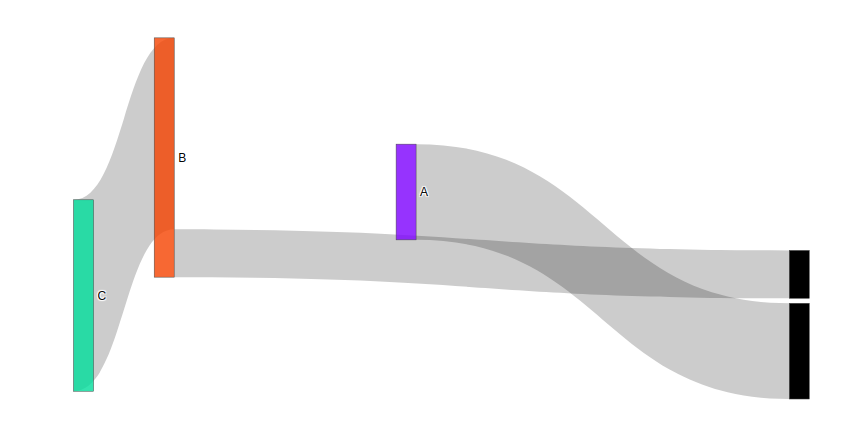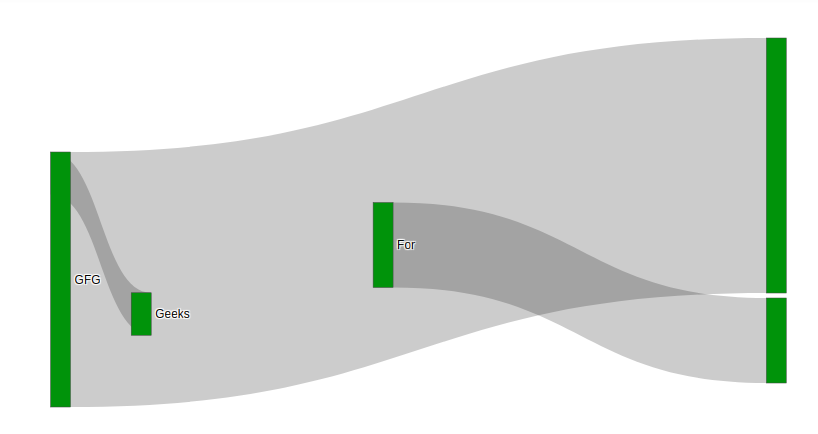Open in App
Not now

# Define Node position in Sankey Diagram in plotly

• Last Updated : 15 Feb, 2022

Plotly is a Python library which is used to design graphs, especially interactive graphs. It can plot various graphs and charts like histogram, barplot, boxplot, spreadplot and many more. It is mainly used in data analysis as well as financial analysis. plotly is an interactive visualization library.

Sankey Diagram is used to visualize the flow by defining the source node and target node. Value parameters is used to set the flow volume. There are basically arrangement for defining a node position. There are –

• perpendicular
• freeform
• fixed

The node position can be set by setting the node.x and node.y value. let’s see the below example for better understanding.

Example 1:

## Python3

 `import` `plotly.graph_objects as go`` ` ` ` `plot ``=` `go.Figure(go.Sankey(``    ``node ``=` `{``        ``"label"``: [``"A"``, ``"B"``, ``"C"``],``        ``"x"``: [``0.5``, ``0.2``, ``0.1``],``        ``"y"``: [``0.4``, ``0.3``, ``0.7``],``        ``'pad'``:``5``},``    ``link ``=` `{``        ``"source"``: [``1``, ``0``, ``1``],``        ``"target"``: [``2``, ``3``, ``4``],``        ``"value"``: [``4``, ``2``, ``1``]}))`` ` `plot.show()`

Output:Example 2:

## Python3

 `import` `plotly.graph_objects as go`` ` ` ` `plot ``=` `go.Figure(go.Sankey(``    ``node ``=` `{``        ``"label"``: [``"Geeks"``, ``"For"``, ``"Geeks"``, ``"GFG"``],``        ``"x"``: [``0.5``, ``0.2``, ``0.1``, ``0.9``],``        ``"y"``: [``0.6``, ``0.8``, ``0.7``],``        ``"color"``: ``"green"``,``        ``'pad'``:``5``},``    ``link ``=` `{``        ``"source"``: [``3``, ``2``, ``1``],``        ``"target"``: [``5``, ``3``, ``7``],``        ``"value"``: [``6``, ``1``, ``2``]}))`` ` `plot.show()`

Output:My Personal Notes arrow_drop_up